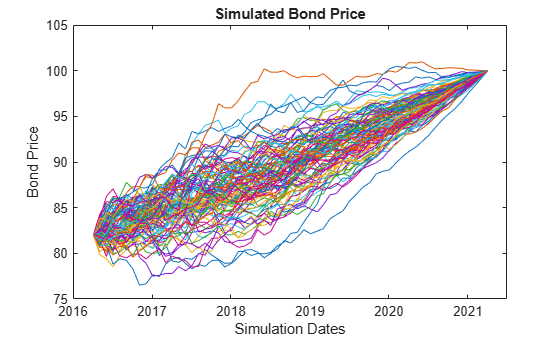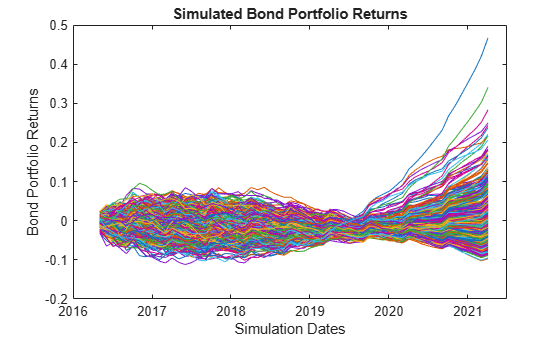# HullWhite1F

Create Hull-White one-factor model

## Description

The Hull-White one-factor model is specified using the zero curve, alpha, and sigma parameters.

Specifically, the HullWhite1F model is defined using the following equations:

`$dr=\left[\theta \left(t\right)-a\left(t\right)r\right]dt+\sigma \left(t\right)dW$`

where:

dr is the change in the short-term interest rate over a small interval.

r is the short-term interest rate.

Θ(t) is a function of time determining the average direction in which r moves, chosen such that movements in r are consistent with today's zero coupon yield curve.

α is the mean reversion rate.

dt is a small change in time.

σ is the annual standard deviation of the short rate.

W is the Brownian motion.

## Creation

### Syntax

``HW1F = HullWhite1F(ZeroCurve,Alpha,Sigma)``

### Description

example

````HW1F = HullWhite1F(ZeroCurve,Alpha,Sigma)` creates a `HullWhite1F` (`HW1F`) object using the required arguments to set the Properties.```

## Properties

expand all

Zero curve, specified as an output from `IRDataCurve` or a `RateSpec` that is obtained from `intenvset`. This is the zero curve used to evolve the path of future interest rates.

Data Types: `object` | `struct`

Mean reversion, specified either as a scalar or function handle which takes time as input and returns a scalar mean reversion value.

Data Types: `double`

Volatility, specified either as a scalar or function handle which takes time as input and returns a scalar mean volatility.

Data Types: `double`

## Object Functions

 `simTermStructs` Simulate term structures for Hull-White one-factor model

## Examples

collapse all

Create a Hull-White one-factor model using an `IRDataCurve`.

```Settle = datenum('15-Dec-2007'); CurveTimes = [1:5 7 10 20]'; ZeroRates = [.01 .018 .024 .029 .033 .034 .035 .034]'; CurveDates = daysadd(Settle,360*CurveTimes,1); irdc = IRDataCurve('Zero',Settle,CurveDates,ZeroRates); alpha = .1; sigma = .01; HW1F = HullWhite1F(irdc,alpha,sigma)```
```HW1F = HullWhite1F with properties: ZeroCurve: [1x1 IRDataCurve] Alpha: @(t,V)inAlpha Sigma: @(t,V)inSigma ```

Use the `simTermStructs` method with the `HullWhite1F` model to simulate term structures.

`SimPaths = simTermStructs(HW1F, 10,'nTrials',100);`

Create a Hull-White one-factor model using a `RateSpec`.

```Settle = datenum('15-Dec-2007'); CurveTimes = [1:5 7 10 20]'; ZeroRates = [.01 .018 .024 .029 .033 .034 .035 .034]'; CurveDates = daysadd(Settle,360*CurveTimes,1); RateSpec = intenvset('Rates',ZeroRates,'EndDates',CurveDates,'StartDate',Settle); alpha = .1; sigma = .01; HW1F = HullWhite1F(RateSpec,alpha,sigma)```
```HW1F = HullWhite1F with properties: ZeroCurve: [1x1 IRDataCurve] Alpha: @(t,V)inAlpha Sigma: @(t,V)inSigma ```

Use the `simTermStructs` method with the `HullWhite1F` model to simulate term structures.

`SimPaths = simTermStructs(HW1F, 10,'nTrials',100);`

Define the zero curve data.

```Settle = datenum('4-Apr-2016'); ZeroTimes = [3/12 6/12 1 5 7 10 20 30]'; ZeroRates = [0.033 0.034 0.035 0.040 0.042 0.044 0.048 0.0475]'; ZeroDates = datemnth(Settle,ZeroTimes*12); RateSpec = intenvset('StartDates', Settle,'EndDates', ZeroDates, 'Rates', ZeroRates)```
```RateSpec = struct with fields: FinObj: 'RateSpec' Compounding: 2 Disc: [8x1 double] Rates: [8x1 double] EndTimes: [8x1 double] StartTimes: [8x1 double] EndDates: [8x1 double] StartDates: 736424 ValuationDate: 736424 Basis: 0 EndMonthRule: 1 ```

Define the bond parameters.

```Maturity = datemnth(Settle,12*5); CouponRate = 0;```

Define the Hull-White parameters.

```alpha = .1; sigma = .01; HW1F = HullWhite1F(RateSpec,alpha,sigma)```
```HW1F = HullWhite1F with properties: ZeroCurve: [1x1 IRDataCurve] Alpha: @(t,V)inAlpha Sigma: @(t,V)inSigma ```

Define the simulation parameters.

```nTrials = 100; nPeriods = 12*5; deltaTime = 1/12; SimZeroCurvePaths = simTermStructs(HW1F, nPeriods,'nTrials',nTrials,'deltaTime',deltaTime); SimDates = datemnth(Settle,1:nPeriods);```

Preallocate and initialize for the simulation.

```SimBondPrice = zeros(nPeriods+1,nTrials); SimBondPrice(1,:,:) = bondbyzero(RateSpec,CouponRate,Settle,Maturity); SimBondPrice(end,:,:) = 100;```

Compute the bond values for each simulation date and path, note that you can vectorize over the trial dimension.

```for periodidx=1:nPeriods-1 simRateSpec = intenvset('StartDate',SimDates(periodidx),'EndDates',... datemnth(SimDates(periodidx),ZeroTimes*12),'Rates',squeeze(SimZeroCurvePaths(periodidx+1,:,:))); SimBondPrice(periodidx+1,:) = bondbyzero(simRateSpec,CouponRate,SimDates(periodidx),Maturity); end plot([Settle SimDates],SimBondPrice) datetick ylabel('Bond Price') xlabel('Simulation Dates') title('Simulated Bond Price')```Define the zero curve data.

```Settle = datenum('4-Apr-2016'); ZeroTimes = [3/12 6/12 1 5 7 10 20 30]'; ZeroRates = [-0.01 -0.009 -0.0075 -0.003 -0.002 -0.001 0.002 0.0075]'; ZeroDates = datemnth(Settle,ZeroTimes*12); RateSpec = intenvset('StartDates', Settle,'EndDates', ZeroDates, 'Rates', ZeroRates)```
```RateSpec = struct with fields: FinObj: 'RateSpec' Compounding: 2 Disc: [8x1 double] Rates: [8x1 double] EndTimes: [8x1 double] StartTimes: [8x1 double] EndDates: [8x1 double] StartDates: 736424 ValuationDate: 736424 Basis: 0 EndMonthRule: 1 ```

Define the bond parameters for the five bonds in the portfolio.

```Maturity = datemnth(Settle,12*5); % All bonds have the same maturity CouponRate = [0.035;0.04;0.02;0.015;0.042]; % Different coupon rates for the bonds nBonds = length(CouponRate);```

Define the Hull-White parameters.

```alpha = .1; sigma = .01; HW1F = HullWhite1F(RateSpec,alpha,sigma)```
```HW1F = HullWhite1F with properties: ZeroCurve: [1x1 IRDataCurve] Alpha: @(t,V)inAlpha Sigma: @(t,V)inSigma ```

Define the simulation parameters.

```nTrials = 1000; nPeriods = 12*5; deltaTime = 1/12; SimZeroCurvePaths = simTermStructs(HW1F, nPeriods,'nTrials',nTrials,'deltaTime',deltaTime); SimDates = datemnth(Settle,1:nPeriods);```

Preallocate and initialize for the simulation.

```SimBondPrice = zeros(nPeriods+1,nBonds,nTrials); SimBondPrice(1,:,:) = repmat(bondbyzero(RateSpec,CouponRate,Settle,Maturity)',[1 1 nTrials]); SimBondPrice(end,:,:) = 100; [BondCF,BondCFDates,~,CFlowFlags] = cfamounts(CouponRate,Settle,Maturity); BondCF(CFlowFlags == 4) = BondCF(CFlowFlags == 4) - 100; SimBondCF = zeros(nPeriods+1,nBonds,nTrials);```

Compute bond values for each simulation date and path. Note that you can vectorize over the trial dimension.

```for periodidx=1:nPeriods if periodidx < nPeriods simRateSpec = intenvset('StartDate',SimDates(periodidx),'EndDates',... datemnth(SimDates(periodidx),ZeroTimes*12),'Rates',squeeze(SimZeroCurvePaths(periodidx+1,:,:))); SimBondPrice(periodidx+1,:,:) = bondbyzero(simRateSpec,CouponRate,SimDates(periodidx),Maturity); end simidx = SimDates(periodidx) == BondCFDates; SimCF = zeros(1,nBonds); SimCF(any(simidx,2)) = BondCF(simidx); ReinvestRate = 1 + SimZeroCurvePaths(periodidx+1,1,:); SimBondCF(periodidx+1,:,:) = bsxfun(@times,bsxfun(@plus,SimBondCF(periodidx,:,:),SimCF),ReinvestRate); end```

Compute the total return series.

`TotalCF = SimBondPrice + SimBondCF;`

Assume the bond portfolio is equally weighted and plot the simulated bond portfolio returns.

```TotalCF = squeeze(sum(TotalCF,2)); TotRetSeries = bsxfun(@rdivide,TotalCF(2:end,:),TotalCF(1,:)) - 1; plot(SimDates,TotRetSeries) datetick ylabel('Bond Portfolio Returns') xlabel('Simulation Dates') title('Simulated Bond Portfolio Returns')```expand all

 Brigo, D. and F. Mercurio. Interest Rate Models - Theory and Practice. Springer Finance, 2006.

 Hull, J. Options, Futures, and Other Derivatives. Prentice-Hall, 2011.